Contents >> Applied Mathematics >> Matrix Algebra >> Principles of Matrix Calculation >> Triangular matrices

 Matrix Algebra - Triangular matrices Triangular matrices The square matrix is called triangular if its elements costing above (below) the main diagonal are equal to zero. For example, the matrix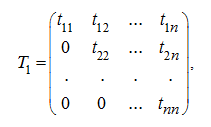where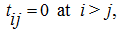is the upper triangular matrix , and the matrix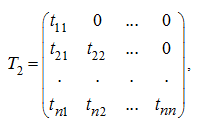where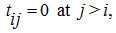is the lower triangular matrix . The diagonal matrix is a special case of a triangular matrix (both upper, and lower). Apparently, that the determinant of a triangular matrix is equal to product of its diagonal elements , that is in our examples: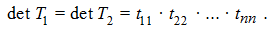Therefore the triangular matrix is regular only in the case when all its diagonal elements are not equal to zero. The sum and product of triangular matrices of the same dimension and identical structure (that is, they are upper, or they are lower) are also triangular matrices of the same dimension and structure. An inverse matrix of a regular triangular matrix is also a triangular matrix of the same dimension and structure. Proceeding from it, the inversion of a triangular matrix does not cause any difficulties.

 < Previous Contents Next >